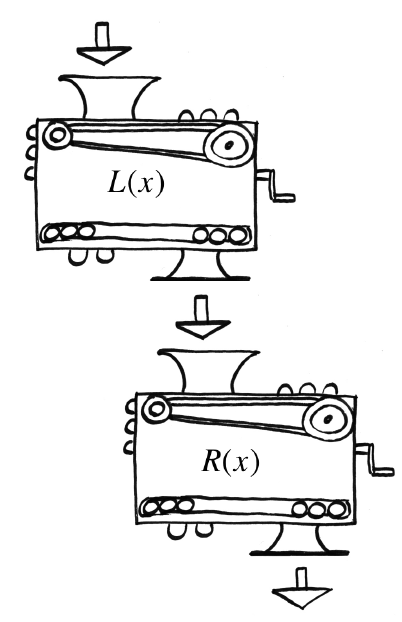### Home > INT3 > Chapter 5 > Lesson 5.1.2 > Problem5-29

5-29.Lacey and Richens each have a personal function machine. Lacey’s machine, $L(x)$, squares the input and then subtracts $1$. Richens’ function machine, $R(x)$, adds $2$ to the input and then multiplies the result by $3$.

1. Write equations that represent $L(x)$ and $R(x)$.

$R(x) = 3(x + 2)$

2. Lacey and Richens decide to connect their two machines so that Lacey’s output becomes Richens’ input. If $3$ is the initial input, what is the final output?

$30$

3. What if the order of the machines is changed? Would it change the output? Justify your answer.

Try computing the result for $x = 3$ if the order is reversed.